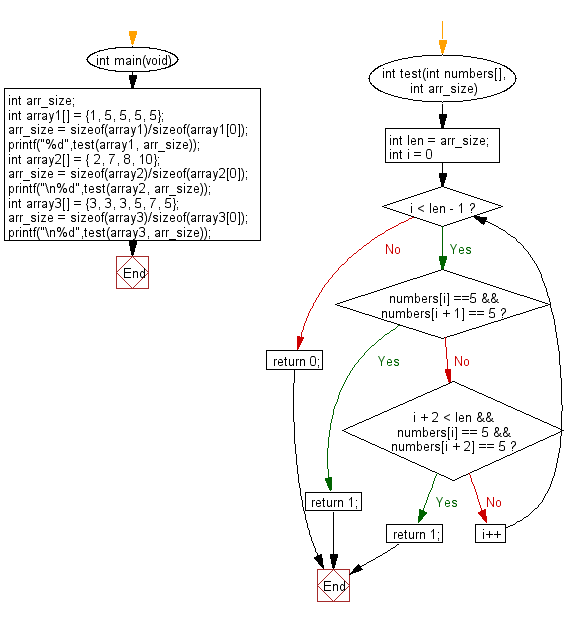﻿ C exercises: Check a given array of integers and return true if the given array contains two 5's next to each other, or two 5 separated by one element - w3resource# C Exercises: Check a given array of integers and return true if the given array contains two 5's next to each other, or two 5 separated by one element

## C-programming basic algorithm: Exercise-61 with Solution

Write a C program to check a given array of integers and return true if the given array contains two 5's next to each other, or two 5 separated by one element.

C Code:

``````#include <stdio.h>
#include <stdlib.h>
int main(void){
int arr_size;
int array1[] = {1, 5, 5, 5, 5};
arr_size = sizeof(array1)/sizeof(array1);
printf("%d",test(array1, arr_size));
int array2[] = { 2, 7, 8, 10};
arr_size = sizeof(array2)/sizeof(array2);
printf("\n%d",test(array2, arr_size));
int array3[] = {3, 3, 3, 5, 7, 5};
arr_size = sizeof(array3)/sizeof(array3);
printf("\n%d",test(array3, arr_size));
}
int test(int numbers[], int arr_size)
{
int len = arr_size;
for (int i = 0; i < len - 1; i++)
{
if (numbers[i] ==5 && numbers[i + 1] == 5) return 1;
if (i + 2 < len && numbers[i] == 5 && numbers[i + 2] == 5) return 1;
}
return 0;
}
``````

Sample Output:

```1
0
1
```

Pictorial Presentation:Flowchart:C Programming Code Editor: